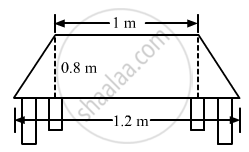Advertisement Remove all ads

# Top Surface of a Table is Trapezium in Shape. Find Its Area If Its Parallel Sides Are 1 M and 1.2 M and Perpendicular Distance Between Them is 0.8 M. - Mathematics

Sum

Top surface of a table is trapezium in shape. Find its area if its parallel sides are 1 m and 1.2 m and perpendicular distance between them is 0.8 m.Advertisement Remove all ads

#### Solution

The given figure is:Lengths of the parallel sides are 1.2 m and 1 m and the perpendicular distance between them is 0.8 m.

∴ Area of the trapezium shaped surface $=\frac{1}{2}\times(\text{ Sum of the parallel sides })\times(\text{ Perpendicular distance })$

$= \frac{1}{2} \times (1 . 2 + 1) \times (0 . 8)$

$=\frac{1}{2}\times2.2\times0.8$

${=0.88 m}^2$

Is there an error in this question or solution?
Advertisement Remove all ads

#### APPEARS IN

RD Sharma Class 8 Maths
Chapter 20 Mensuration - I (Area of a Trapezium and a Polygon)
Exercise 20.2 | Q 10 | Page 23
Advertisement Remove all ads

#### Video TutorialsVIEW ALL 

Advertisement Remove all ads
Share
Notifications

View all notifications

Forgot password?
Course